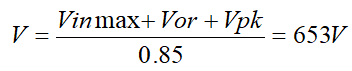### 教你计算输入电容及DCM、CCM、QR变压器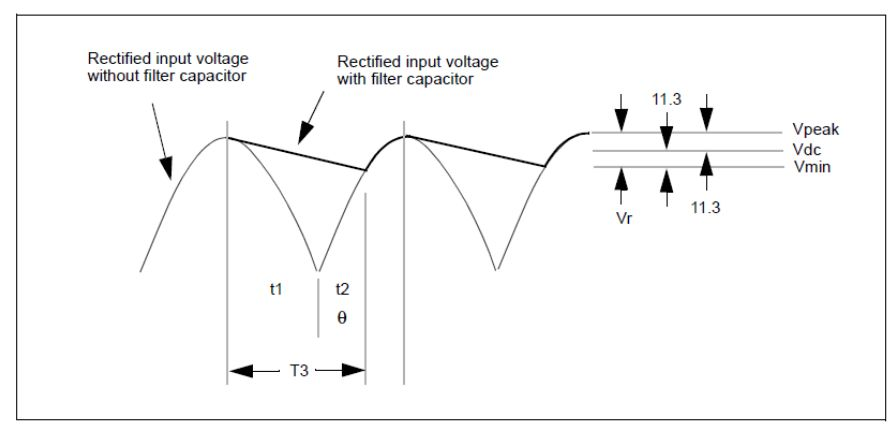Vpk=90*1.414=127V

Vmin=Vdc-(Vpk-Vdc)=103V

Idc*T3=C*△V

△V=Vpk-Vmin=127-103=24V

Vx=Vpksinθx，根据已知条件，Vx=103V，Vpk=127V，可以得到θx=54度，所以t2=54*10ms/180=3mS， T3=t1+t2=8mS。

C=1.7*8/24=0.57mF=570uF

1）DCM变压器设计过程：

Vdc*Dmax=Vor*(1-Dmax)，

n=Vor/(Vo+Vf)=3.32   Vf为整流二极管压降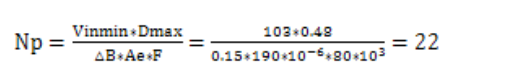Ns=Np/n=6.32，选择7匝，

Np=3.32*7=23匝

Po=0.5L*I*I*F/η  I=Vinmin*Dmax/(L*F)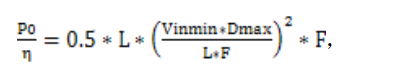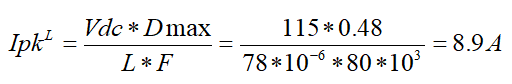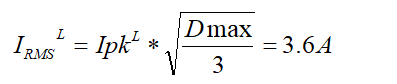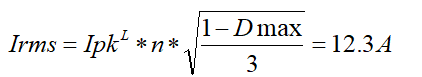Vdc*Dmax=Vor*(1-Dmax)，

n=Vor/(Vo+Vf)=3.32   Vf为整流二极管压降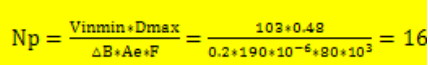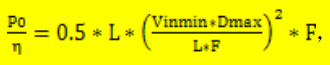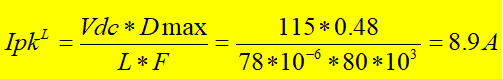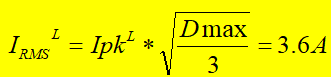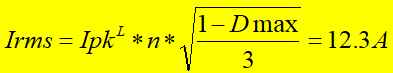2）CCM变压器设计过程：

CCM变压器的设计，必须首先确定一个负载点，在该状态下，变压器工作在BCM状态下，如果负载继续增加则进入CCM，如果负载减小，则进入DCM，一般情况下，我会选择zui低输入电压下额定负载的70%为BCM状态。

70%负载情况下，输出功率为P0.7=27.6*6*0.7=116W，因此峰值电流为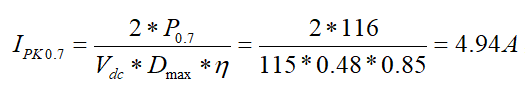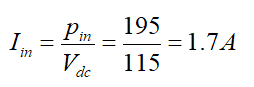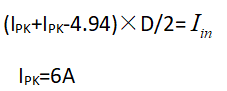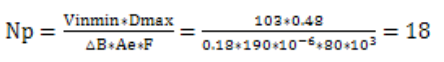根据伏秒平衡，可以得到以下公式，

Vdc*Dmax=Vor*(1-Dmax)，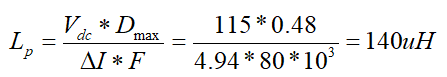3）QR模式变压器的设计过程

zui低输入电压103V，zui大占空比Dmax选择0.48，在zui低输入电压情况下，变压器工作在临界模式（QR模式实际只是近似与临界模式不是在临界模式，只是为了方便计算，用临界模式来近似计算），则根据伏秒平衡

Vdc*Dmax=Vor*(1-Dmax)，

Vor= Vdc*Dmax/(1-Dmax)

=103*0.48/（1-0.48）

=95V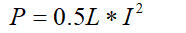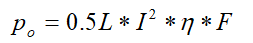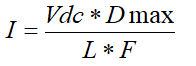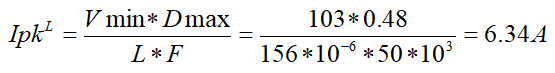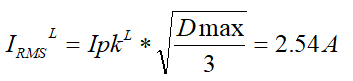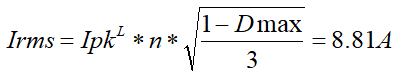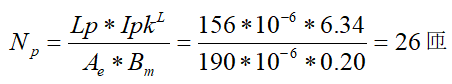MOS管的选择